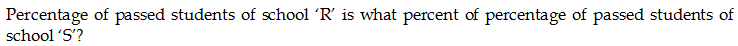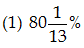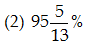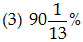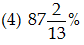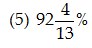## PREPARATORY QUESTIONS

### DATA INTERPRETATION (TABLE)

#### PREPARATORY SET-41 (TABLE)

Direction (Qs.1 to 5): Given table shows the ratio of passed students, failed students and absent students in final exams of six different schools in 2017.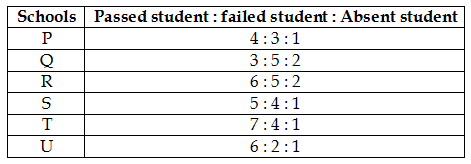Note: Total students in any school = passed students + failed students + absent students

Question No : 1

If total students in school ‘P’ is equal to the total students in school ‘T’ and absent students of school ‘T’ is 32 then, find the difference between passed students of school ‘P’ and passed students of school ‘T’?

(1) 64

(2) 48

(3) 36

(4) 28

(5) 32

Question No : 2

Failed students from school ‘U’ is what percent of the failed students from school ‘R’ if absent students from both schools are equal?

(1) 60%

(2) 66(2/3)%

(3) 48%

(4) 80%

(5) 90%

Question No : 3

If ratio of total students from school Q, R and S is 3 : 2 : 4 and absent students of school Q are 312 then find the average of total number of students in school Q, R and S together.

(1) 1560

(2) 1340

(3) 1440

(4) 2080

(5) 1040

Question No : 4

If passed students from school ‘P’ is equal to the failed students of school ‘U’, then absent students of school ‘P’ is what percent of the absent students of school ‘U’?

(1) 40%

(2) 80%

(3) 50%

(4) 30%

(5) 60%

Question No : 5